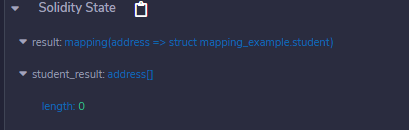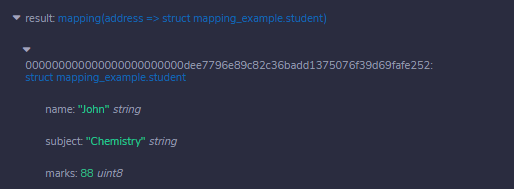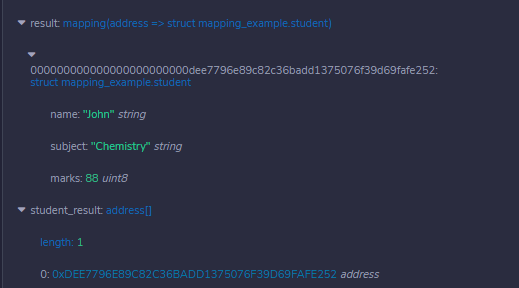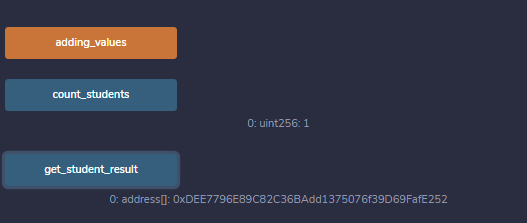Solidity – Mappings

• Difficulty Level : Basic
• Last Updated : 13 Jul, 2020

Mapping in Solidity acts like a hash table or dictionary in any other language. These are used to store the data in the form of key-value pairs, a key can be any of the built-in data types but reference types are not allowed while the value can be of any type. Mappings are mostly used to associate the unique Ethereum address with the associated value type.

Syntax:

mapping(key => value) <access specifier> <name>;

Creating a Mapping

Mapping is defined as any other variable type, which accepts a key type and a value type.

Example: In the below example, the contract mapping_example a structure is defined and mapping is created.

Solidity

 // Solidity program to // demonstrate mappingpragma solidity ^0.4.18;    // Defining contract contract mapping_example {          //Defining structure    struct student     {        // Declaring different         // structure elements        string name;        string subject;        uint8 marks;    }          // Creating a mapping    mapping (    address => student) result;    address[] public student_result;    }

Output :As the mapping is created let’s try to add some values to the mapping for better understanding.

Example: In the below example, the contract mapping_example defines a structure, mapping is created and values are added to the mapping.

Solidity

 // Solidity program to // demonstrate adding // values to mappingpragma solidity ^0.4.18;   // Creating contractcontract mapping_example {          //Defining structure     struct student {          //Declaring different         // structure elements        string name;        string subject;        uint8 marks;    }      // Creating mapping    mapping (      address => student) result;    address[] public student_result;          // Function adding values to     // the mapping    function adding_values() public {        var student           = result[0xDEE7796E89C82C36BAdd1375076f39D69FafE252];          student.name = "John";        student.subject = "Chemistry";        student.marks = 88;        student_result.push(          0xDEE7796E89C82C36BAdd1375076f39D69FafE252) -1;      }      }

Output :Getting values

We have added values to the mapping, to retrieve the values we have to create a function that returns the values added to the mapping.

Example: In the below example, the contract mapping_example defines a structure, mapping is created, values are added to the mapping, and values are retrieved from the mapping.

Solidity

 // Solidity program to// demonstrate retrieve// values from the mapping  pragma solidity ^0.4.18;    contract mapping_example {          // Defining Structure    struct student {          // Declaring different data types        string name;        string subject;        uint8 marks;    }          // Creating mapping    mapping (      address => student) result;    address[] student_result;         // Function adding values to the mapping    function adding_values() public {        var student           = result[0xDEE7796E89C82C36BAdd1375076f39D69FafE252];          student.name = "John";        student.subject = "Chemistry";        student.marks = 88;        student_result.push(          0xDEE7796E89C82C36BAdd1375076f39D69FafE252) -1;      }           // Function to retrieve      // values from a mapping     function get_student_result(     ) view public returns (address[]) {        return student_result;    }}

Output :Counting Mappings

Mappings can be counted so that we can know how many values are stored in mapping.

Example: In the below example, the contract mapping_example defines a structure, mapping is created, values are added to the mapping, and values are retrieved from the mapping.

Solidity

 // Solidity program to// count number of // values in a mappingpragma solidity ^0.4.18;    contract mapping_example {          // Defining structure    struct student {          // Declaring different        // structure elements        string name;        string subject;        uint8 marks;    }          // Creating mapping    mapping (address => student) result;    address[] student_result;          //Function adding values to the mapping    function adding_values() public {        var student          = result[0xDEE7796E89C82C36BAdd1375076f39D69FafE252];          student.name = "John";        student.subject = "Chemistry";        student.marks = 88;        student_result.push(          0xDEE7796E89C82C36BAdd1375076f39D69FafE252) -1;      }           // Function to retrieve      // values from the mapping     function get_student_result(     ) view public returns (address[]) {        return student_result;    }      // Function to count number     // of values in a mapping    function count_students(    ) view public returns (uint) {        return student_result.length;    }}

Output :My Personal Notes arrow_drop_up